### Understand TRIM Function and Acknowledge Examples

In this lesson you can learn how to use TRIM function.

Thanks to TRIM function you can remove spaces from the text.

Syntax of TRIM function is:

=TRIM(text)

If you have text with extra spaces in A1 cell, just writhe a formula =TRIM(A1). TRIM function remove extra spaces which you doesn’t need.

You can also use TRIM function with other functions.

### Remove extra spaces in Excel

To remove spaces you don’t need just use TRIM function. Use this simple formula.

=TRIM(A1)### Extract text after the last full stop in a text string

You want to extract text after the last string. Just use the formula below with TRIM function.

=TRIM(RIGHT(SUBSTITUTE(A1,”.”,REPT(” “,10)),10))Of course you can modify this formula. If you have problem with other character (eg. question mark) just change full stop with this character in formula.

=TRIM(RIGHT(SUBSTITUTE(A1,”?”,REPT(” “,10)),10))

If your string text is longer than 10 just increase it.

=TRIM(RIGHT(SUBSTITUTE(A1,”?”,REPT(” “,1000)),1000))

### Finding word in a long sting of text

You want to find some word in a long string of text and you don’t know how to do it? Try this formula:

=TRIM(LEFT(SUBSTITUTE(MID(A1,FIND(“WORD”,A1&”WORD”),1000),”*”,REPT(” “,1000)),1000))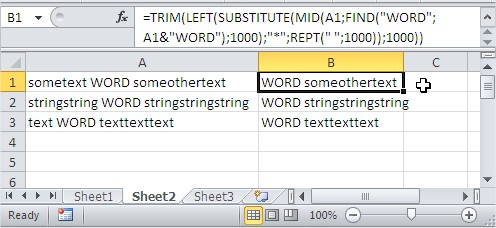Just replace WORD to your text.

If you want to get a text without WORD string just change formula to:

=TRIM(LEFT(SUBSTITUTE(MID(A1;FIND(“WORD”;A1&”WORD”)+5;1000);”*”;REPT(” “;1000));1000))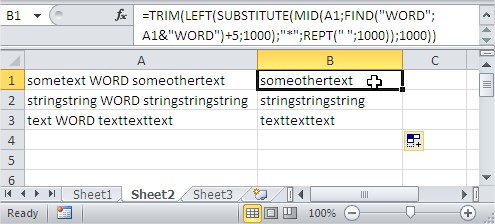These 2 above formulas should work also without TRIM function. Use them with TRIM function to work better and always fine.

### Find exact value in cell

Task is to find exact value in the cell. It means that when you want to find ’15’, Excel will find you only cells with ’15’, but not with ‘1515’, ‘215’, ‘150’…

=IF(ISNUMBER(FIND(“;15;”,”;”&TRIM(A1)&”;”)),15,””)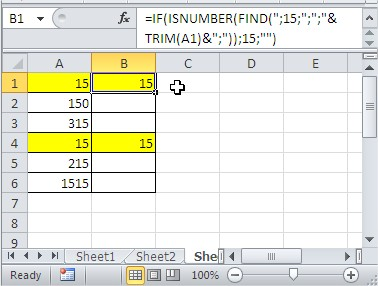### Simple Trim Formula

This formula is to trim a specific text on Microsoft Excel.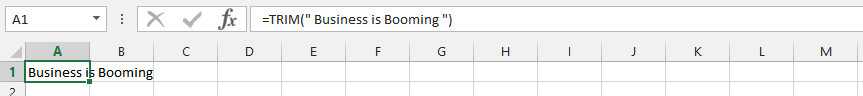### TRIM with Multiple Space Issues

This example focuses on using the trim function with multiple spacing issues.### Trim a Cell

This example focuses on trimming texts that is in a cell.### Trim and Find

This example focuses on trimming texts in multiple cells. It is under the circumstances that we’d have the words layout, and the texts we want to use are in different cells.### TRIM and Flexibilities

We have text in different cells, but we want to add something to it. This example focuses on trimming both cells and text.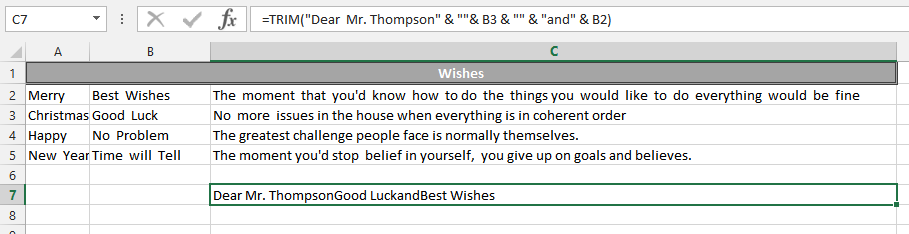### Trim and Left

This is the example that focuses on trimming and using data to the left. It is the acknowledgment of the letter in left, using trim and left functions simultaneously.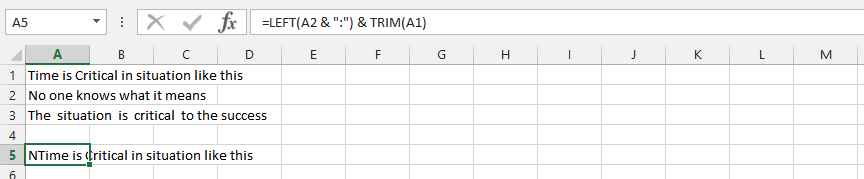### Trim, Right, Substitute and Rept.

This example uses four different functions – trim, right, substitute and rept. – to find a solid result with the simultaneous usage of the function.### Trim, Left, Substitute, Mid, Find and Rept.

This example uses six different functions – trim, left, substitute, mid, find and rept. – to get a specific result. It is being used to eliminate some texts from the row.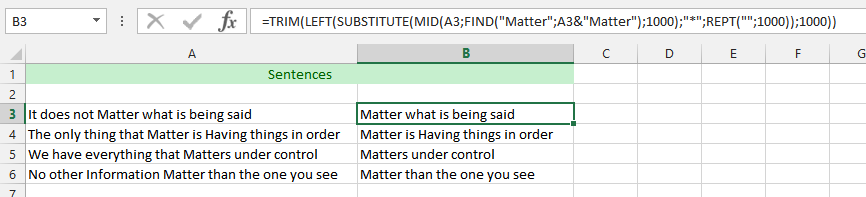### Previous Example Excluding “Matter”

This example use the very same data as previous example, but this time we are excluding the word “matter” from the spreadsheet. The best part is, we are using the very same functions as the previous example – trim, left, rept., substitute, mid and find.### IF, ISNUMBER, FIND and TRIM

This is the example that make use of four different functions – IF, ISNUMBER, FIND and TRIM – to see if we would get a different result.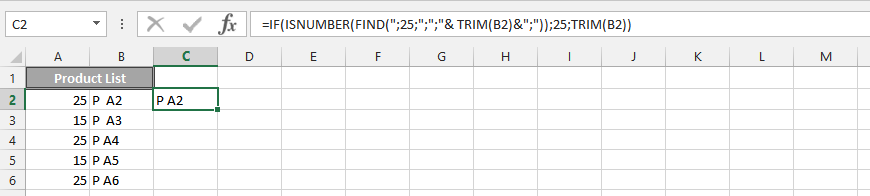## Template

```Further reading: